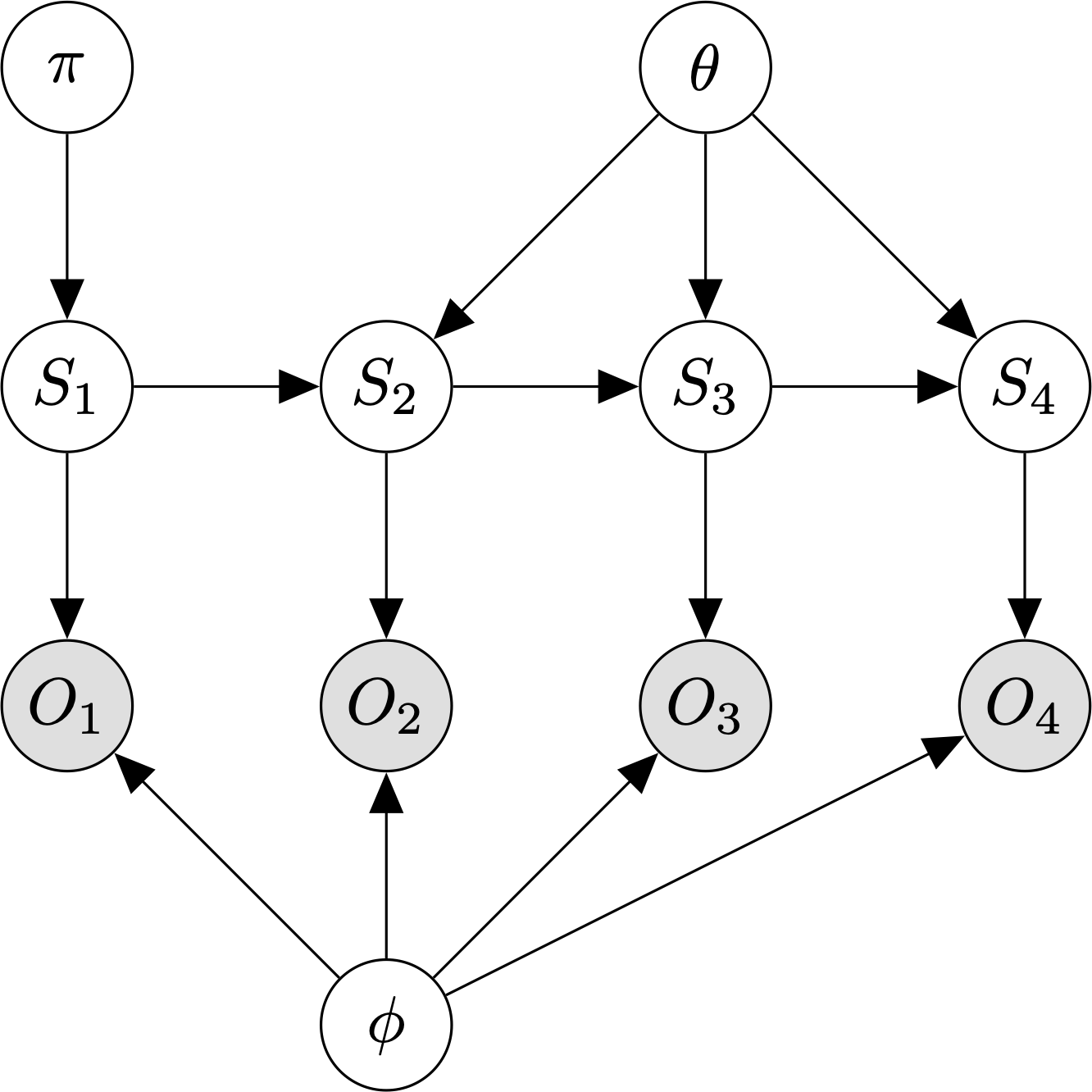# Hidden Markov models¶

A hidden Markov model (HMM) is a generative model for sequences of observations.Graphical model for an HMM with $$T=4$$ timesteps.

An HMM assumes:

1. The observations, O, are generated by a process whose states, $$S$$, are hidden from the observer.
2. Each hidden state is a discrete random variable.
3. The hidden state at time t is independent of all hidden states before time $$t - 1$$.
4. The observation $$O_t$$ is independent of all previous states and observations given the current hidden state, $$S_t$$.

The parameters of an HMM model are:

• $$\pi$$, the prior specifying $$P(S_1)$$.
• $$\theta$$, the $$K \times K$$ transition matrix specifying $$P(S_t \mid S_{t-1})$$.
• $$\phi$$, the output model defining $$P(Y_t \mid S_t)$$. If the observations are discrete, this is a $$K \times L$$ emission matrix, where L is the number of unique observation symbols.

The HMM joint distribution of a sequence of states and observations is:

$P(S_{1:T}, O_{1:T}) = P(S_1) P(O_1 \mid S_1) \prod_{t=2}^T P(S_t \mid S_{t-1})P(O_t \mid S_t)$

where $$X_{1:T}$$ is shorthand for $$X_1, \ldots, X_T$$.

As with other latent-variable models, we use the expectation-maximization (EM) algorithm to learn the model parameters. The HMM-optimized version of the EM algorithm is known as the forward-backward / Baum-Welch algorithm.

Models

References

  Ghahramani, Z. (2001). “An Intro to HMMs and Bayesian networks”. International Journal of Pattern Recognition and AI, 15(1): 9-42.